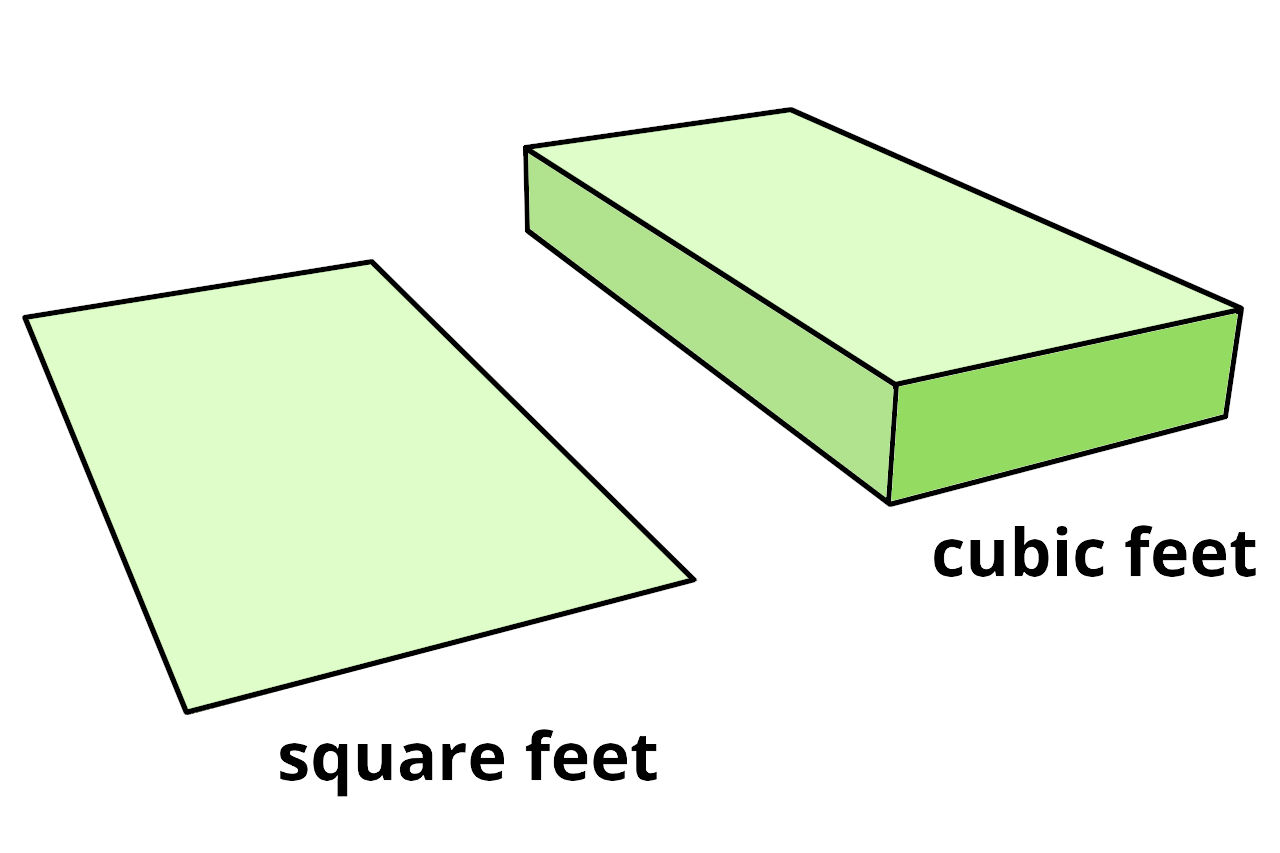# Square Feet to Cubic Feet Calculator

Use the calculator below to convert square feet to cubic feet by entering the area and height. You can also convert cubic feet to square feet by entering the volume and height.

## Result:

Cubic Feet
Learn how we calculated this below

## How to Convert Square Feet to Cubic Feet

Before we start performing any calculations, it’s essential to understand the difference between square feet and cubic feet. Square footage is a measure of area, while cubic footage is a measure of volume.

The units of area are square lengths, such as ft2 or in2, and the units of volume are cubic lengths, such as ft3 or in3.

The image below shows the difference between the two measurements.Because these are completely different types of measurements, you’ll need some additional data to calculate one from the other. In order to convert either way, you’ll need the height of the space or object.

To convert square feet to cubic feet using the area and height, you’ll need to use the conversion formula below.

### Square Ft to Cubic Ft Formula

You can use the following formula to calculate cubic feet from square feet and height:

cu ft = sq ft × height

The volume in cubic feet is equal to the area in square feet times the height in feet. If you first need to calculate square feet, try our square footage calculator to find the area.

For example, let’s calculate the cubic footage of a 5 square foot area that is 2 feet high.

volume = 5 sq ft × 2 ft
volume = 10 cu ft

You can also use our calculator to convert square feet to cubic yards.

## How to Convert Cubic Feet to Square Feet

When you know the volume in cubic feet and the height, you can rearrange the formula above to calculate square feet.

### Cubic Ft to Square Ft Formula

This formula shows how to calculate an area from a volume.

sq ft = cu ft ÷ height

The area in square feet is equal to the volume in cubic feet divided by the height in feet. Check out our cubic feet calculator to find the volume of a space.

For example, let’s calculate the square footage of an area in a 15 cubic foot volume that is 3 feet high.

area = 15 cu ft ÷ 3 ft
area = 5 sq ft

You might also be interested in our linear feet to square feet calculator.